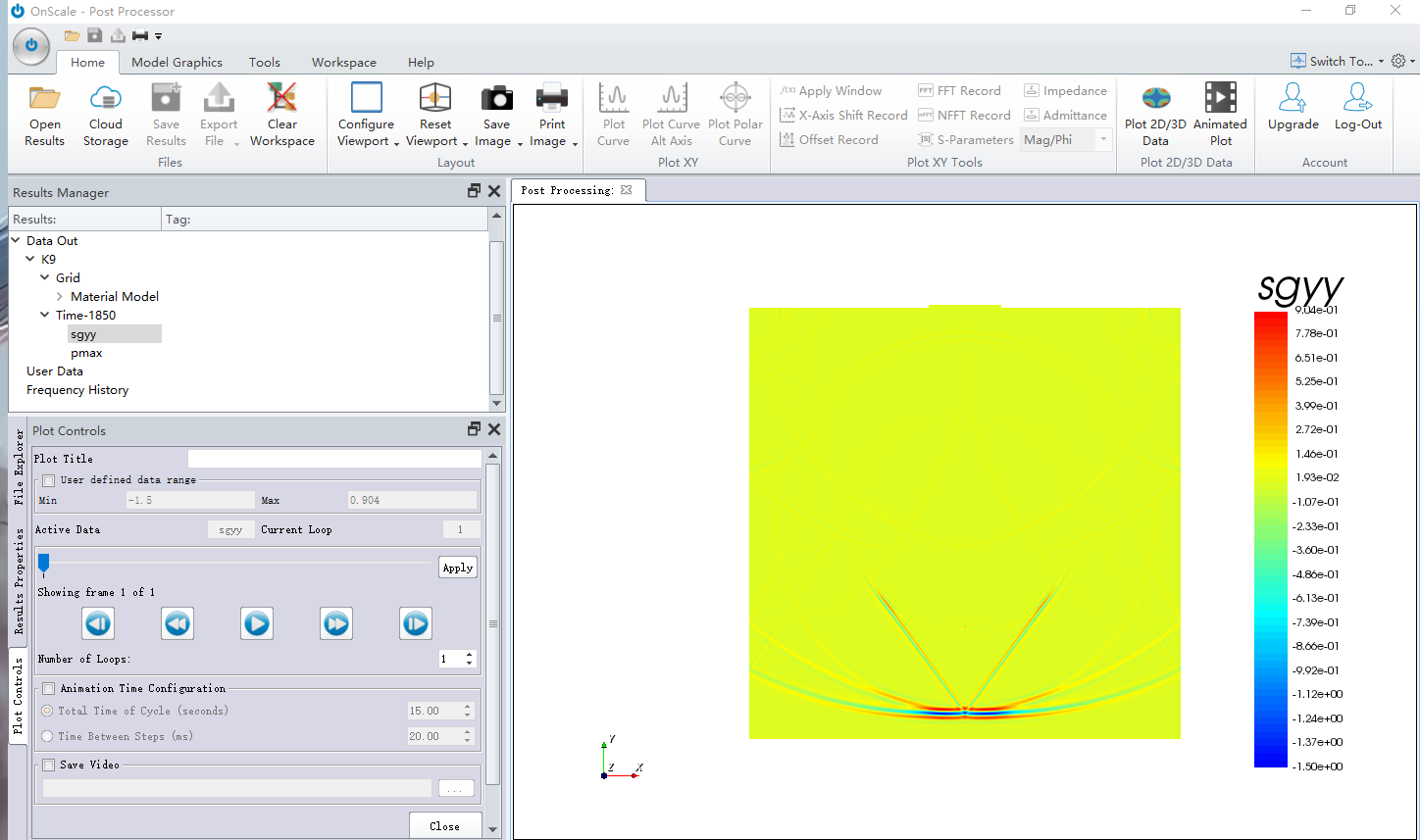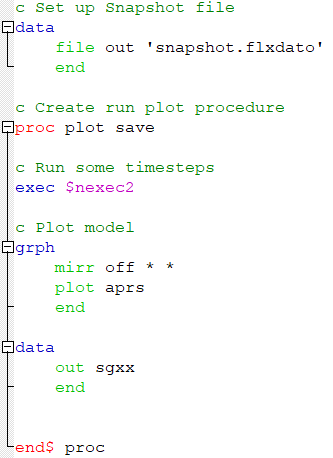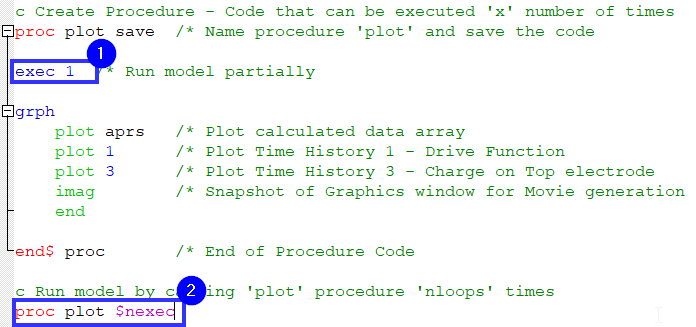# How to output the stress ‘sgyy’ at every moment Answered

Hi，

The following are sections of my simulation code. I openthe flex dato file and plot the sgyy data-array，but it only shows the stress of the final moment.

`calc	strs sgyy 	pres acoustic	max aprs * pmax         end`
`data      out sgyy      out modl      out pmax      end`

I wanna get the stress data at every moment，how to  code？• You can output a snapshot of any array like this.

1. You open a new file 'snapshot.flxdato' you can call it whatever you like.
2. Then in the PROC procedure you add the line DATA OUT SGXX this will output stress at every loop in the simulation.If you want to do it for every timestep (moment) you would need to edit the code like so:

1. Change to exec 1 this will execute 1 timestep
2. Most of our example scripts have the variable \$nexec which stores the total number of timesteps for a simulation so you would use this variable as the number of times to run the model for.I wouldn't recommend this however. For example in our PZT_2D disc the total number of timesteps calculated is 2410 this would mean the file would containe 2410 snapshots of that array. The file size could get quite large especially if you are running a 3D model.